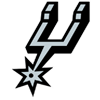2021 NBA MVP Odds
+140
1.4 to 1Stephen Curry
33.7% implied probability

+475
4.75 to 1Kevin Durant
14.1% implied probability

+700
7 to 1Giannis Antetokounmpo
10.1% implied probability

+1300
13 to 1Nikola Jokic
5.8% implied probability

+2200
22 to 1Luka Doncic
3.5% implied probability

+3500
35 to 1Joel Embiid
2.2% implied probability

+4000
40 to 1Trae Young
2.0% implied probability

+4000
40 to 1Devin Booker
2.0% implied probability

+4000
40 to 1Paul George
2.0% implied probability

+4000
40 to 1Jimmy Butler
2.0% implied probability

+4500
45 to 1James Harden
1.8% implied probability

+5000
50 to 1DeMar DeRozan
1.6% implied probability

+6000
60 to 1Damian Lillard
1.3% implied probability

+6000
60 to 1Jayson Tatum
1.3% implied probability

+6000
60 to 1Chris Paul
1.3% implied probability

+6000
60 to 1LeBron James
1.3% implied probability

+6000
60 to 1Anthony Davis
1.3% implied probability

+6500
65 to 1Zach LaVine
1.2% implied probability

+7500
75 to 1LaMelo Ball
1.1% implied probability

+7500
75 to 1Donovan Mitchell
1.1% implied probability

+8000
80 to 1Ja Morant
1.0% implied probability

+12000
120 to 1Karl-Anthony Towns
0.7% implied probability

+15000
150 to 1Rudy Gobert
0.5% implied probability

+15000
150 to 1Julius Randle
0.5% implied probability

+15000
150 to 1Bam Adebayo
0.5% implied probability

+15000
150 to 1Bradley Beal
0.5% implied probability

+25000
250 to 1Russell Westbrook
0.3% implied probability

+25000
250 to 1Anthony Edwards
0.3% implied probability

+25000
250 to 1Jaylen Brown
0.3% implied probability

+30000
300 to 1Domantas Sabonis
0.3% implied probability

+40000
400 to 1Lonzo Ball
0.2% implied probability

+50000
500 to 1Fred VanVleet
0.2% implied probability

+50000
500 to 1Malcolm Brogdon
0.2% implied probability

+50000
500 to 1Pascal Siakam
0.2% implied probability

+50000
500 to 1Deandre Ayton
0.2% implied probability

+50000
500 to 1Zion Williamson
0.2% implied probability

+50000
500 to 1John Collins
0.2% implied probability

+50000
500 to 1Shai Gilgeous-Alexander
0.2% implied probability

+50000
500 to 1De'Aaron Fox
0.2% implied probability

+50000
500 to 1Kristaps Porzingis
0.2% implied probability

+50000
500 to 1Khris Middleton
0.2% implied probability

+50000
500 to 1Kyrie Irving
0.2% implied probability

+50000
500 to 1Ben Simmons
0.2% implied probability

+50000
500 to 1Christian Wood
0.2% implied probability

+50000
500 to 1D'Angelo Russell
0.2% implied probability

+50000
500 to 1Kyle Lowry
0.2% implied probability

+50000
500 to 1Clint Capela
0.2% implied probability

+50000
500 to 1CJ McCollum
0.2% implied probability

+50000
500 to 1Dejounte Murray
0.2% implied probability

+50000
500 to 1Draymond Green
0.2% implied probability

+50000
500 to 1Nikola Vucevic
0.2% implied probability

+50000
500 to 1Tobias Harris
0.2% implied probability

+50000
500 to 1Jrue Holiday
0.2% implied probability

+100000
1000 to 1Cade Cunningham
0.1% implied probability

+100000
1000 to 1Tyrese Haliburton
0.1% implied probability

+100000
1000 to 1Brandon Ingram
0.1% implied probability

+100000
1000 to 1Kemba Walker
0.1% implied probability

+100000
1000 to 1Aaron Gordon
0.1% implied probability

+100000
1000 to 1Jerami Grant
0.1% implied probability

+100000
1000 to 1Norman Powell
0.1% implied probability

+100000
1000 to 1Cole Anthony
0.1% implied probability